# Math in Focus Grade 4 Chapter 6 Answer Key Fractions and Mixed Numbers

Practice the problems of Math in Focus Grade 4 Workbook Answer Key Chapter 6 Fractions and Mixed Numbers to score better marks in the exam.

## Math in Focus Grade 4 Chapter 6 Answer Key Fractions and Mixed Numbers

Math Journal

Is the model correct? If not, explain why it is wrong. Draw the correct model.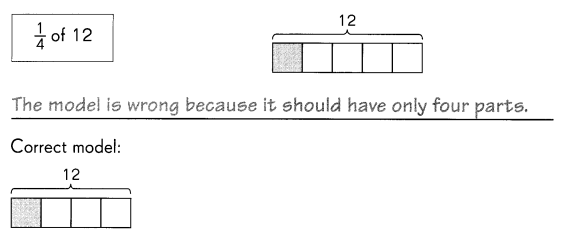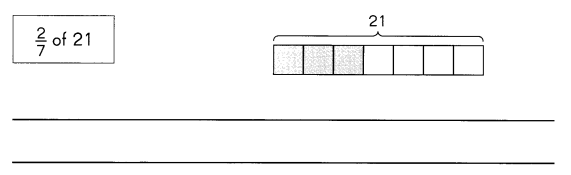Correct model: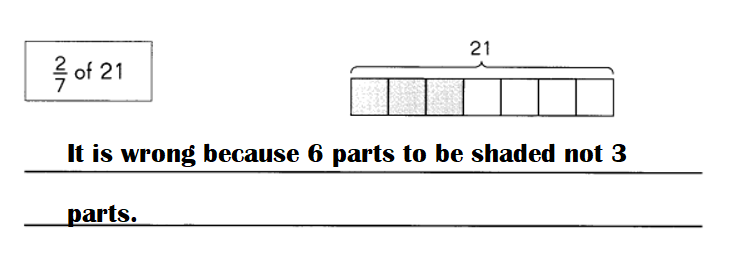Explanation:
(2 ÷ 7 ) × 21 is wrong because 6 parts should be shaded not 3.
(2 ÷ 7 ) × 21 = 2 × 3 = 6.

Challenging Practice
Question 1.
Show 1$$\frac{1}{4}$$– shaded, if 1 whole is made up of 4 squares. Some of the shading has been done for you.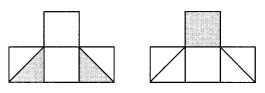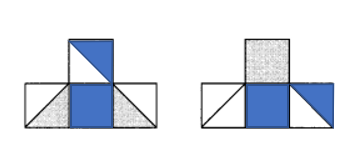Explanation:
1$$\frac{1}{4}$$  of 4
= (5 ÷ 4) × 4
= 5.

Question 2.
Is the answer of 21 × $$\frac{2}{7}$$ the same as that of 2 × $$\frac{21}{7}$$? Show your work.
Yes, both the answers are same.

Explanation:
21 × $$\frac{2}{7}$$ = 3 × 2 = 6.
2 × $$\frac{21}{7}$$ = 2 × 3 = 6.

Question 3.
Write a fraction and a whole number that have the same product as the problem below.
8 × $$\frac{3}{4}$$ = ___
____ × _____ = ___
8 × $$\frac{3}{4}$$ = 3 × $$\frac{8}{4}$$ = 3 × 2 = 6.

Explanation:
8 × $$\frac{3}{4}$$ = 2 × 3 = 6.
3 × $$\frac{8}{4}$$ = 3 × 2 = 6.

Problem Solving
Caroline places five poles A, B, C, D, and E in order along a straight line. The distance between poles A and D is 1 yard. The distance between poles B and C is the same as the distance between poles A and B.
Poles A and B are $$\frac{1}{5}$$ yard apart.
The distance between D and E is $$\frac{7}{10}$$ yard.
How far apart are poles B and E?
Distance between poles B and E = 3 ÷ 2 or $$\frac{3}{2}$$.

Explanation:
Distance between poles A and D = 1 yard.
The distance between poles B and C is the same as the distance between poles A and B.
=> Distance between poles B and C = Distance between poles A and B.
Let the distance between poles B and C be X.
Distance between poles A and B = $$\frac{1}{5}$$ yard.
Distance between poles D and E = $$\frac{7}{10}$$ yard.

Distance between poles A and B + Distance between poles B and C + Distance between C and D = Distance between poles A and D
= $$\frac{1}{5}$$ + $$\frac{1}{5}$$ + Distance between C and D = 1
= $$\frac{2}{5}$$ + Distance between C and D = 1
= Distance between C and D = 1 – $$\frac{2}{5}$$
= Distance between C and D = (5 – 2) ÷ 5
= Distance between C and D = 3 ÷ 5 or $$\frac{3}{5}$$ yard.

Distance between poles B and E = Distance between poles B and C + Distance between C and D + Distance between poles D and E
= $$\frac{1}{5}$$ + $$\frac{3}{5}$$ + $$\frac{7}{10}$$
= $$\frac{4}{5}$$ + $$\frac{7}{10}$$
= (8 + 7) ÷ 10
= 15 ÷ 10
= 3 ÷ 2 or $$\frac{3}{2}$$